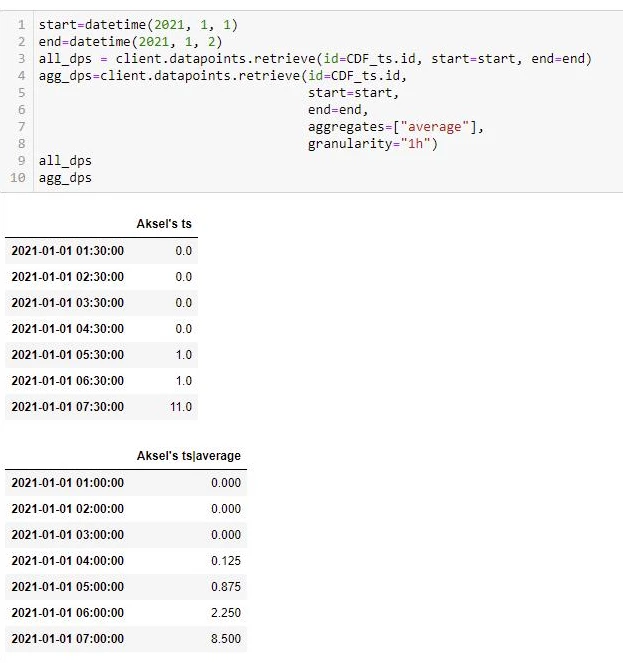Solved

# Timeseries aggregates gives strange results for "average"

• 4 replies
• 208 views

Userlevel 1• Contributor
• 5 repliesAksels ts is Original data , ts average at 04:00 = 0.125, can only be explained by interpolated values

The example above shows that 1h aggregates of type average computed at time-point 03:00 takes into considereation the interpolated values in the time-span 03:00 to 04:00. Is it always like this as long as i choose granualarity 1h ?
I could not find the answer to my question here:
Aggregation | Cognite documentation

icon

Best answer by Håkon V. Treider 3 May 2021, 23:15

I am not entirely sure I get your question, but I’ll try to answer as best as I can!The timestamps for aggregates are labelled by the left boundary. That means the period from 03:00 (inclusive) to 04:00 (exclusive) will be labelled as 03:00.

In your data above, for the aggregate data point at 03:00, with the value 0.0 is zero because all datapoints inside and the two outside points (for the purpose of interpolation to the boundary) are zero.

The aggregate data point, at 04:00, with the value 0.125 can be computed as follows:

1. From 04:00 to 04:30 the value is 0.0, so this part of the interval does not contribute to the final aggregate value.
2. However, in order to find the contribution from the timespan from 04:30 to 05:00, we need to use linear interpolation to find the value at the right boundary, as the documentation specifies. The next value is at 05:30, so this would give an in-between value at the right boundary of 0.5. The average is defined as the time-weigted average (mean distance from 0), so we integrate to find the value/area of this right-angled triangle:
30 (minutes, “triangle length”) * 0.5 (“triangle height”) * 0.5 (formula constant) = 7.5 (minutes).
3. The average is thus the sum of these two contributions divided by the length of the interval:
(0 + 7.5) / 60 = 0.5^3 = 0.125

View original

### 4 replies

Userlevel 2I am not entirely sure I get your question, but I’ll try to answer as best as I can!The timestamps for aggregates are labelled by the left boundary. That means the period from 03:00 (inclusive) to 04:00 (exclusive) will be labelled as 03:00.

In your data above, for the aggregate data point at 03:00, with the value 0.0 is zero because all datapoints inside and the two outside points (for the purpose of interpolation to the boundary) are zero.

The aggregate data point, at 04:00, with the value 0.125 can be computed as follows:

1. From 04:00 to 04:30 the value is 0.0, so this part of the interval does not contribute to the final aggregate value.
2. However, in order to find the contribution from the timespan from 04:30 to 05:00, we need to use linear interpolation to find the value at the right boundary, as the documentation specifies. The next value is at 05:30, so this would give an in-between value at the right boundary of 0.5. The average is defined as the time-weigted average (mean distance from 0), so we integrate to find the value/area of this right-angled triangle:
30 (minutes, “triangle length”) * 0.5 (“triangle height”) * 0.5 (formula constant) = 7.5 (minutes).
3. The average is thus the sum of these two contributions divided by the length of the interval:
(0 + 7.5) / 60 = 0.5^3 = 0.125

Userlevel 1Thanks, I agree with the computed value. The last thing I wonder about is how/if I can be sure that no matter how the original datapoints are distributed with respect to time, the average for hourly aggregates computed at say 01:00 will always be based on the weighted averages over the timespan from [01:00; 02:00[ .

Userlevel 2Thanks, I agree with the computed value. The last thing I wonder about is how/if I can be sure that no matter how the original datapoints are distributed with respect to time, the average for hourly aggregates computed at say 01:00 will always be based on the weighted averages over the timespan from [01:00; 02:00).

Almost yes; the start and end of the interval can be influence by outside points. I made an illustration to show it better, modifying the illustration in the documentation:

Here you have two points inside the interval (green), one point before (red) influencing the part of the average from the left boundary to the first inside datapoint (same and opposite argument for blue / datapoint after the interval).

Userlevel 1Thanks a lot Håkon. We are comparing the measurements from some standard sensors with those from fiscal meters that by design display hourly averages. So, when averaging the measurements from standard meters we needed to know what was done so they are averaged in the same way.